# Chemical Reactions Types of Reactions There are six

• Slides: 20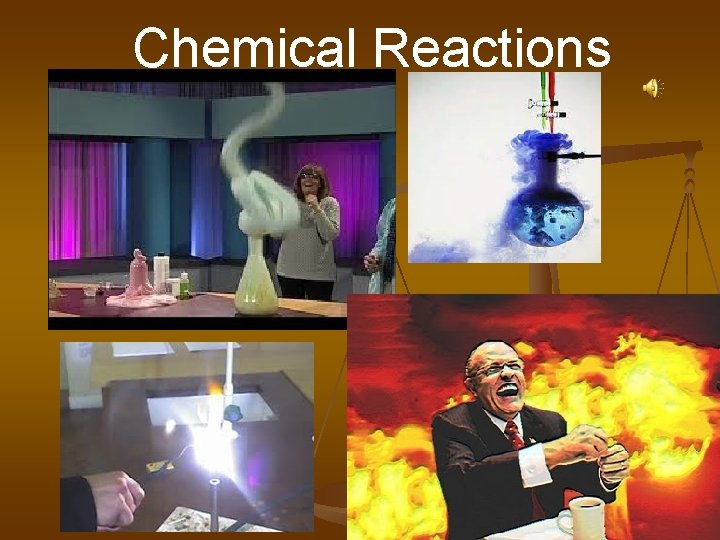Chemical Reactions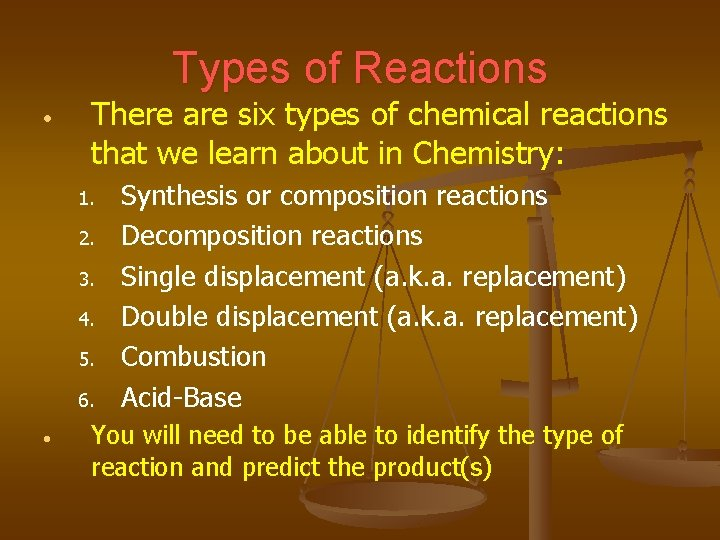Types of Reactions • There are six types of chemical reactions that we learn about in Chemistry: 1. 2. 3. 4. 5. 6. • Synthesis or composition reactions Decomposition reactions Single displacement (a. k. a. replacement) Double displacement (a. k. a. replacement) Combustion Acid-Base You will need to be able to identify the type of reaction and predict the product(s)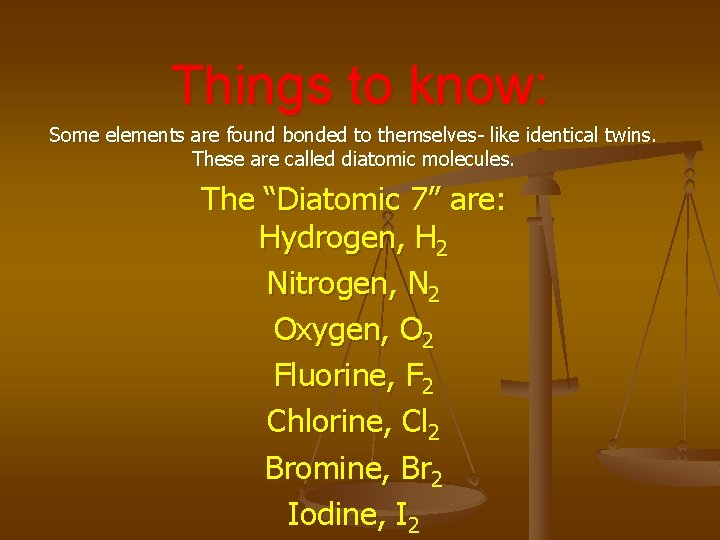Things to know: Some elements are found bonded to themselves- like identical twins. These are called diatomic molecules. The “Diatomic 7” are: Hydrogen, H 2 Nitrogen, N 2 Oxygen, O 2 Fluorine, F 2 Chlorine, Cl 2 Bromine, Br 2 Iodine, I 2The diatomic 7 make a “ 7” starting at element #7, plus HThings to Remember: Follow the ‘rules’ of bonding when writing compounds or molecules For example: How does Sodium bond with Chlorine? Na+1 Cl-1 so it will be: Na. Cl H+1 will bond with O-2 as H 2 O You may be tempted to write compounds and molecules differently to balance an equation- but you can’t! They bond as they bond.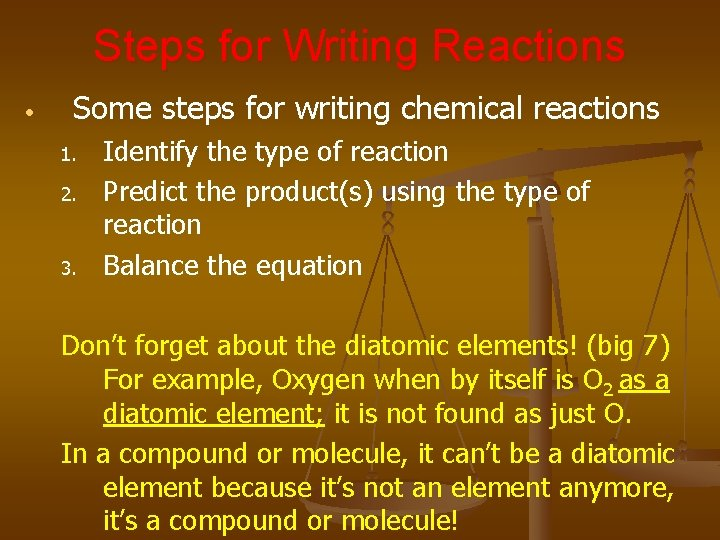Steps for Writing Reactions • Some steps for writing chemical reactions 1. 2. 3. Identify the type of reaction Predict the product(s) using the type of reaction Balance the equation Don’t forget about the diatomic elements! (big 7) For example, Oxygen when by itself is O 2 as a diatomic element; it is not found as just O. In a compound or molecule, it can’t be a diatomic element because it’s not an element anymore, it’s a compound or molecule!Synthesis Reactions • Here is an example of a synthesis reaction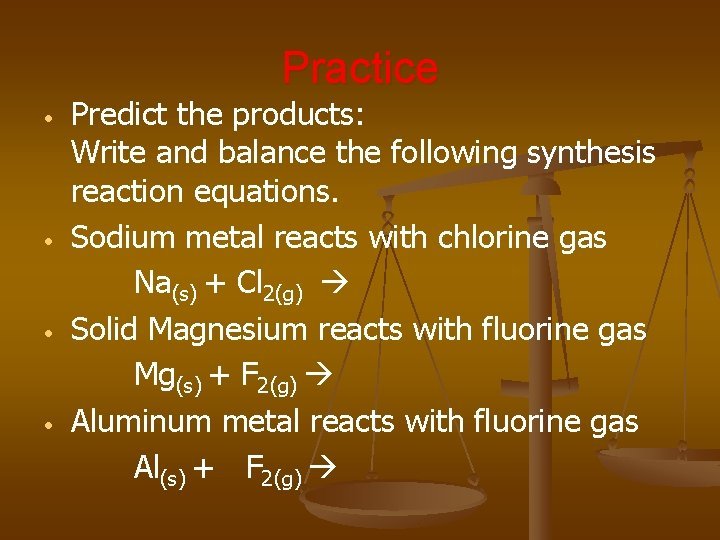Practice • • Predict the products: Write and balance the following synthesis reaction equations. Sodium metal reacts with chlorine gas Na(s) + Cl 2(g) Solid Magnesium reacts with fluorine gas Mg(s) + F 2(g) Aluminum metal reacts with fluorine gas Al(s) + F 2(g)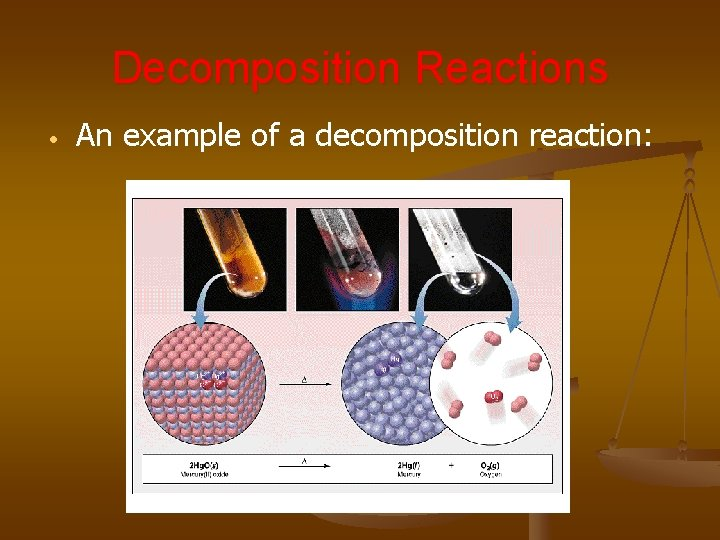Decomposition Reactions • An example of a decomposition reaction:Decomposition Exceptions • Carbonates and chlorates are special case decomposition reactions that do not go to the elements. • Carbonates (CO 32 -) decompose to carbon dioxide and a metal oxide • • Chlorates (Cl. O 3 -) decompose to oxygen gas and a metal chloride • • Example: Ca. CO 3 CO 2 + Ca. O Example: 2 Al(Cl. O 3)3 2 Al. Cl 3 + 9 O 2 There are other special cases, but we will not explore those in Chemistry IPractice • • • Predict the products: Then, write and balance the following decomposition reaction equations: Solid Lead (IV) oxide decomposes Pb. O 2(s) Aluminum nitride decomposes Al. N(s)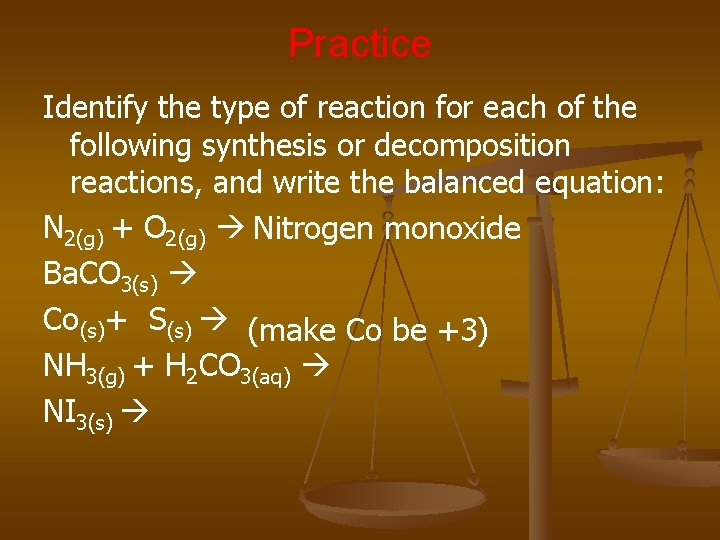Practice Identify the type of reaction for each of the following synthesis or decomposition reactions, and write the balanced equation: N 2(g) + O 2(g) Nitrogen monoxide Ba. CO 3(s) Co(s)+ S(s) (make Co be +3) NH 3(g) + H 2 CO 3(aq) NI 3(s)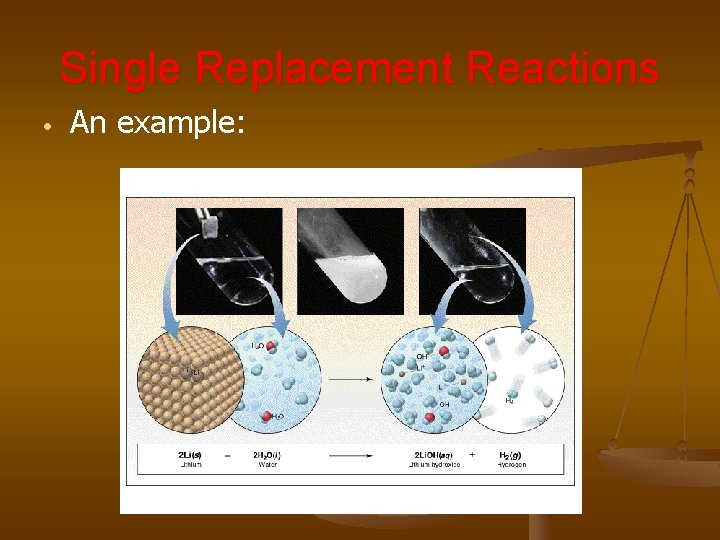Single Replacement Reactions • An example:Single Replacement Reactions • • Write and balance the following single replacement reaction equation: Zinc metal reacts with aqueous hydrochloric acid Zn(s) + HCl(aq) Note: Zinc replaces the hydrogen ion in the reaction… Why?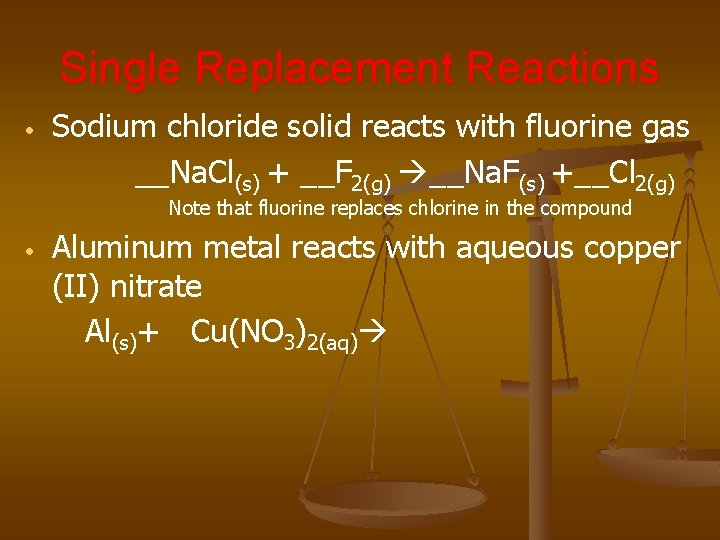Single Replacement Reactions • Sodium chloride solid reacts with fluorine gas __Na. Cl(s) + __F 2(g) __Na. F(s) +__Cl 2(g) Note that fluorine replaces chlorine in the compound • Aluminum metal reacts with aqueous copper (II) nitrate Al(s)+ Cu(NO 3)2(aq)Double Replacement Reactions • • • Think about it like “foil”ing in algebra, the first and last ions go together and the inside ions go together Example: Ag. NO 3(aq) + Na. Cl(s) Ag. Cl(s) + Na. NO 3(aq) Another example: K 2 SO 4(aq) + Ba(NO 3)2(aq)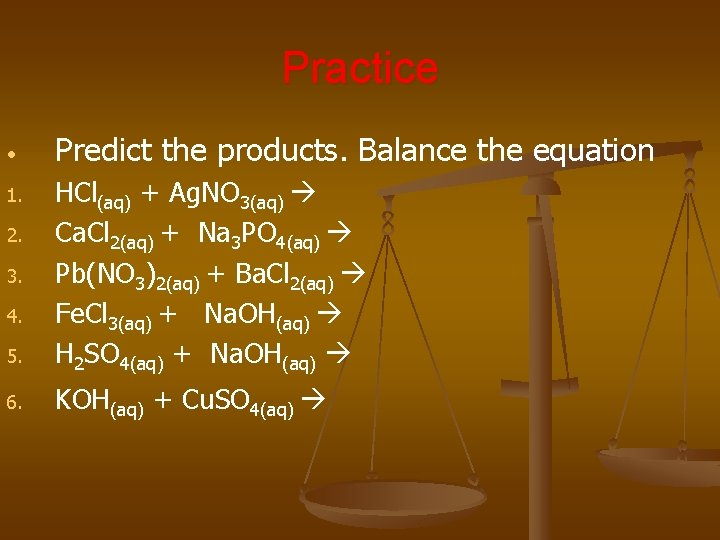Practice • Predict the products. Balance the equation 5. HCl(aq) + Ag. NO 3(aq) Ca. Cl 2(aq) + Na 3 PO 4(aq) Pb(NO 3)2(aq) + Ba. Cl 2(aq) Fe. Cl 3(aq) + Na. OH(aq) H 2 SO 4(aq) + Na. OH(aq) 6. KOH(aq) + Cu. SO 4(aq) 1. 2. 3. 4.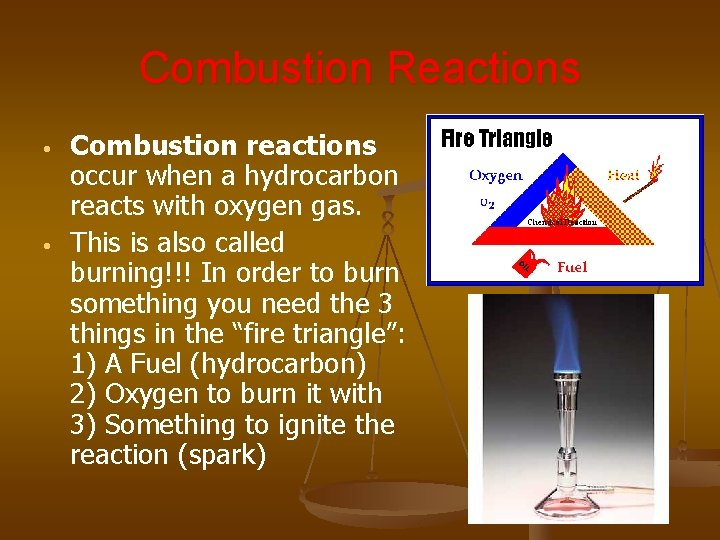Combustion Reactions • • Combustion reactions occur when a hydrocarbon reacts with oxygen gas. This is also called burning!!! In order to burn something you need the 3 things in the “fire triangle”: 1) A Fuel (hydrocarbon) 2) Oxygen to burn it with 3) Something to ignite the reaction (spark)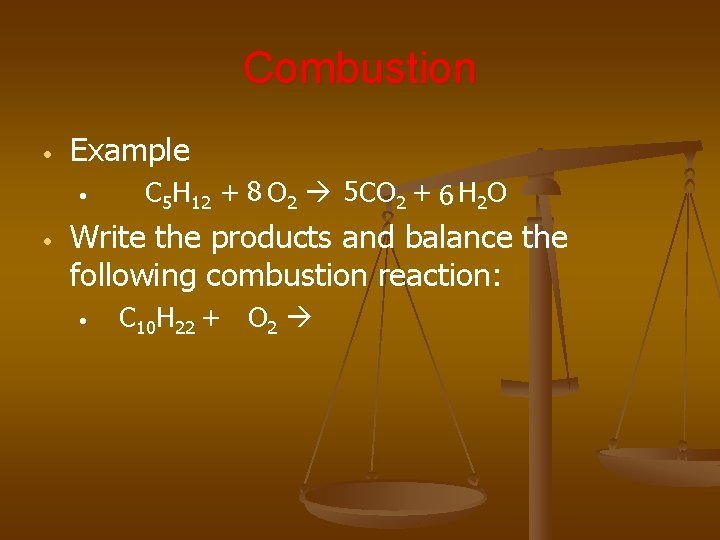Combustion • Example • • C 5 H 12 + 8 O 2 5 CO 2 + 6 H 2 O Write the products and balance the following combustion reaction: • C 10 H 22 + O 2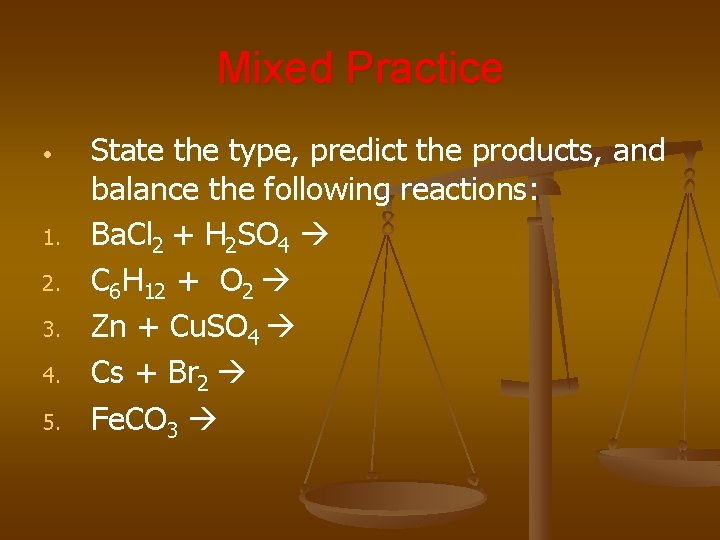Mixed Practice • 1. 2. 3. 4. 5. State the type, predict the products, and balance the following reactions: Ba. Cl 2 + H 2 SO 4 C 6 H 12 + O 2 Zn + Cu. SO 4 Cs + Br 2 Fe. CO 3